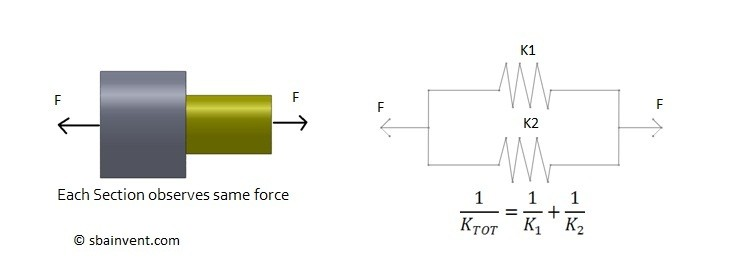# Axial Stiffness

Stiffness is used to correlate the load to the amount the object will deflect do to that load. To find stiffness the equation below would be used.

(Eq 1)  $k=\frac{P}{δ}=\frac{AE}{L}$

P = Force

δ = Deflection

A = Cross-sectional Area

E = Young’s Modulus

k = Stiffness

So when would you be interested in the stiffness? Well for one thing if you are interested in the natural frequency of the part you will need to determine the stiffness of the part. Another case though would be if you are dealing with parts that are made out of different material.

Now to calculate the overall stiffness of a part made out of two different materials you would treat the problem the same way you would to calculate the overall resistance of an electrical circuit.

To start, what if you had to different materials that are connected in parallel? To find the stiffness of the overall part refer to the image below.Now, what if you had two parts of dissimilar material connected in series? To find the overall all stiffness refer to the image below.By determining the overall stiffness of the parts you can now either determine the total force required to deflect that object by a given distance or how much and object will deflect due to a certain force.